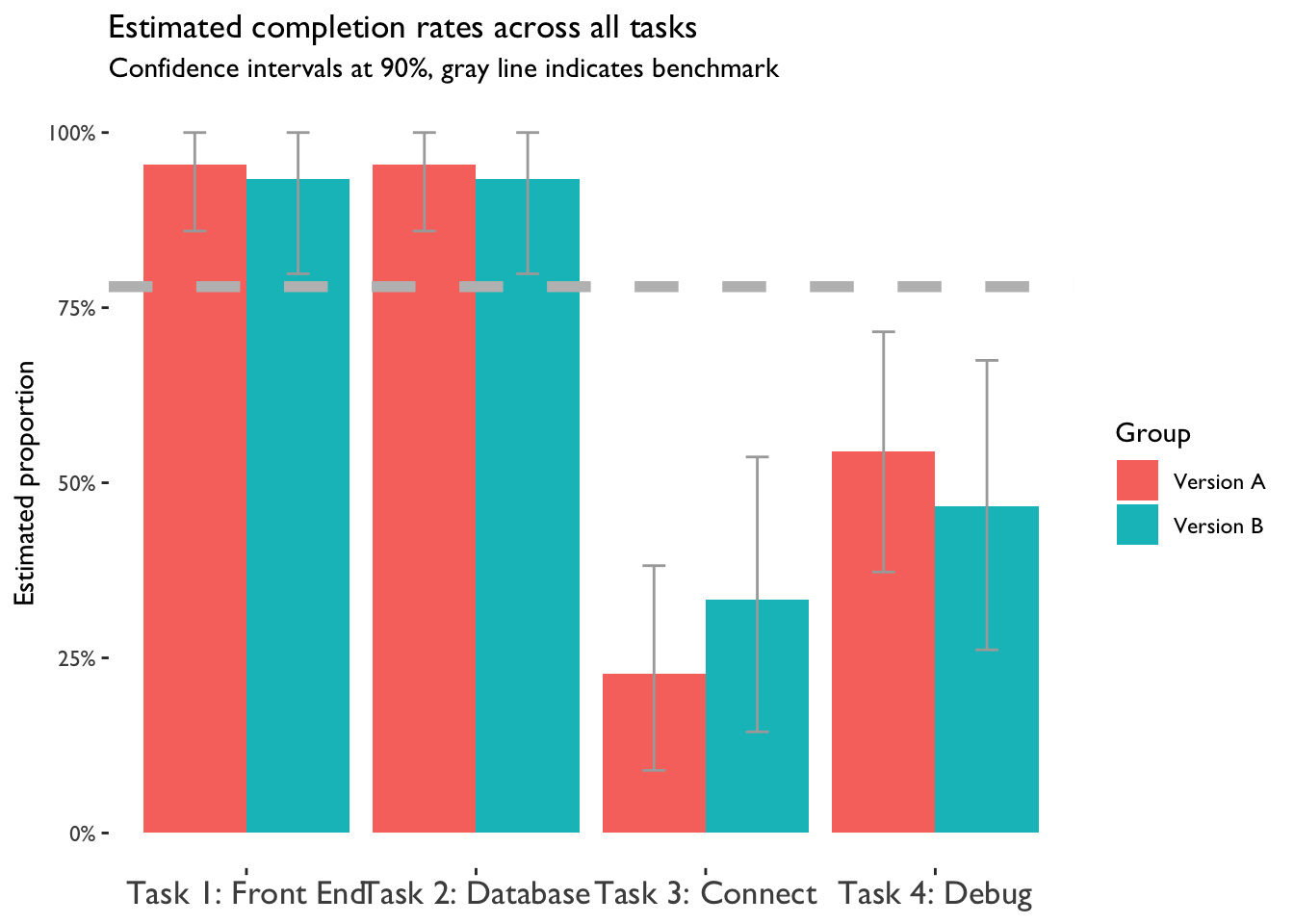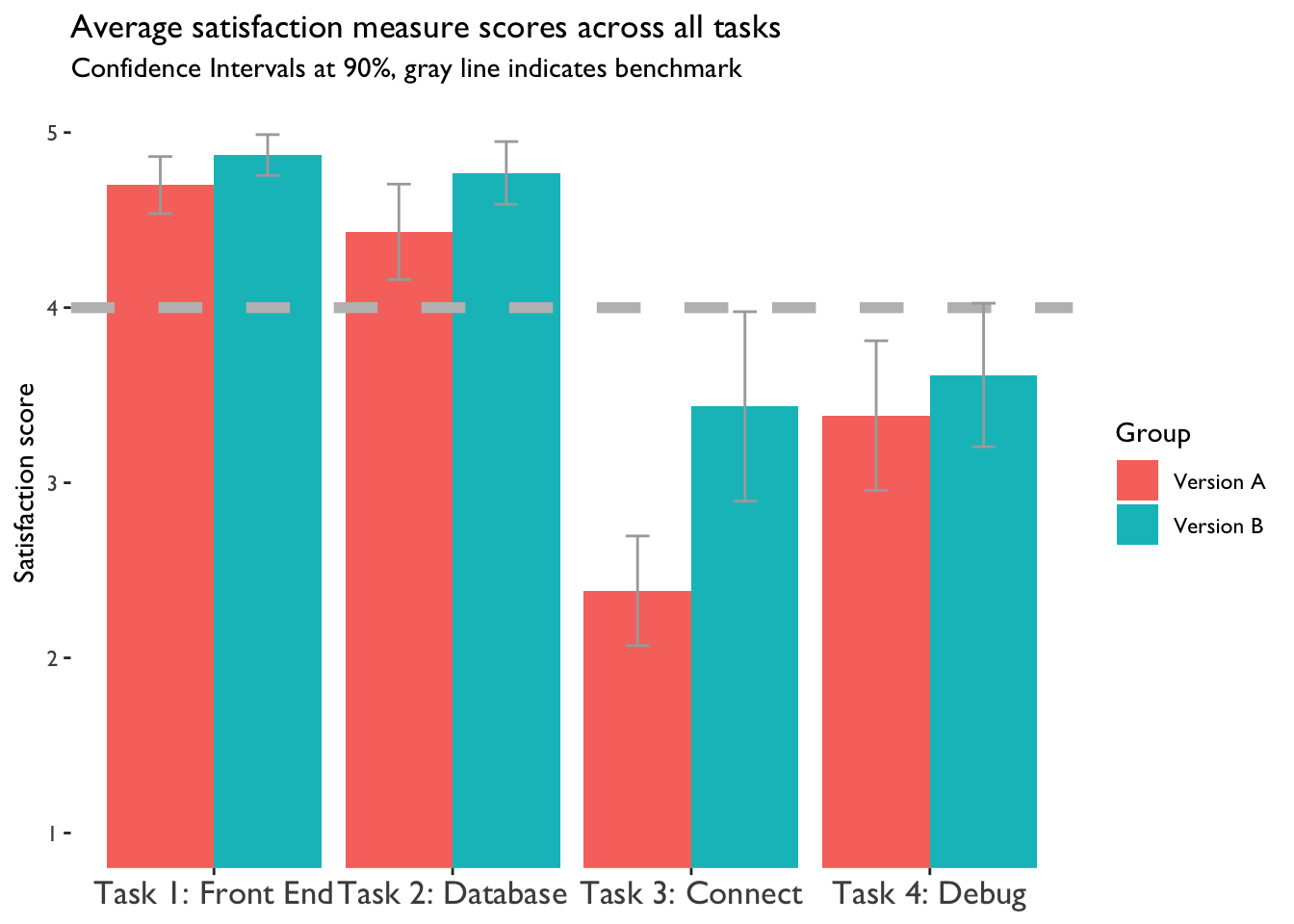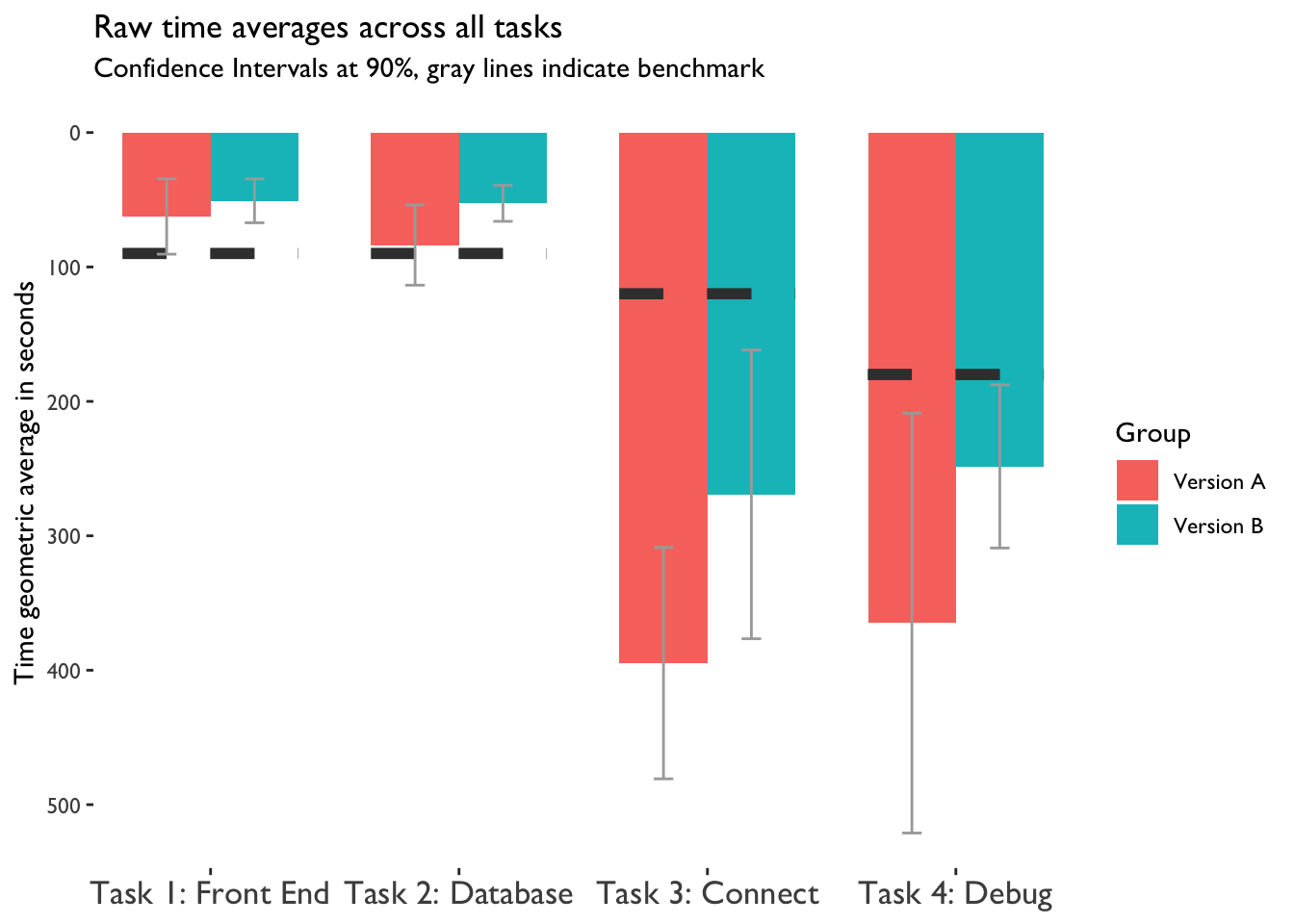### Why should you read this?

This document is about our process and choices in using the Single Usability Metric (SUM) to benchmark our products. Read this if you want to learn about the open source R code and specific calculations to use in your own research.

Our organization wanted an empirical measure of the usability of OpenShift Version A and to make a benchmark comparison with OpenShift Version B. This allowed us to give robust evidence for the effect that our design choices had on the usability of our product.

We chose the Single Usability Metric (SUM) method to standardize and summarize three classic usability metrics: completion rates, time to completion, and subjective satisfaction. Each of these metrics were collected per user across four tasks: add a front end container, add a database container, connect the front end and database, and debug a crashing pod (due to misspelled envrionment variable). The metrics are created the levels of metrics at each task, each task as a whole, and finaly, each version. The output numbers are based on an NPS-like scale of -100 to 100.

To get these behavioral metrics we recruited 33 users of OpenShift: 20 completed our tasks on version Version A and 13 did so on Version B.

Our organization wanted an empirical measure of the usability of OpenShift Version A and to make a benchmark comparison with OpenShift Version A B. This allowed us to give robust evidence for the effect of our design choices a critical components of the user experience.

####The main research question is Do our design choices between versions positively affect usability?

This R Markdown document will walk through the code and results of our research question for the purposes of making the calculation method accessible on free software (R Statistics).

### Load packages and get the data in

``````#packages
library(tidyverse) #we essentially need dplyr and ggplot2``````
``````## Registered S3 methods overwritten by 'ggplot2':
##   method         from
##   [.quosures     rlang
##   c.quosures     rlang
##   print.quosures rlang``````
``## ── Attaching packages ────────────────────────────────────────────────────── tidyverse 1.2.1 ──``
``````## ✔ ggplot2 3.1.1     ✔ purrr   0.3.2
## ✔ tibble  2.1.3     ✔ dplyr   0.8.1
## ✔ tidyr   0.8.3     ✔ stringr 1.4.0
## ✔ readr   1.3.1     ✔ forcats 0.4.0``````
``````## ── Conflicts ───────────────────────────────────────────────────────── tidyverse_conflicts() ──
``library(psych) #this is used to calculate a geometric mean``
``````##
## Attaching package: 'psych'``````
``````## The following objects are masked from 'package:ggplot2':
##
##     %+%, alpha``````
``library(ggthemes) #tufte plots let us maximize our data:ink ratio``

So now we have loaded our packages we will use for calculations and plotting. The essential columns in your long (not wide) dataframe must be, respective to our example: participant (factor/numeric), task (factor), time in seconds (numeric), satisfaction (numeric), completion (numeric), group/version (factor).

``````df <- data_location_local #use your location

head(df) #what do the data look like?``````
``````##   par task time      sat comp group
## 1   3    1   54 5.000000    1     1
## 2   3    2   47 5.000000    1     1
## 3   3    3  362 3.333333    1     1
## 4   3    4  242 5.000000    1     1
## 5   4    1   82 4.666667    1     1
## 6   4    2   78 4.666667    1     1``````

### Precalculation

To calculate confidence intervals, we must choose a critical value. We chose a 90% confidence interval. This means our critical value is as follows:

``zval <- 1.64 #z value at 90% confidence``

Next, we need to choose our benchmarks. For satisfaction, there is a standard value based on past research, which is 4 on a scale out of 1-5. Completion calculations do not use a benchmark (we’ll talk more about this later). Time benchmarks are task specific, and you can read about how to choose them here. A general rule of thumb is the 80-95th percentile fastest time to complete for those who rated satisfaction above 4/5.

``````df\$tspec[df\$task==1] <- 90 #time spec for task 1 in seconds #based on derivation from data, 80th percentile from sat above 4 could be used

## Calculating

Now we have everything in place to start our calculations. We will calculate each metric separately (classic means and SUM standard means) and then bind the dataframes. All of this can be done in vanilla R, but I find dplyr to speed up my process considerably.

### Satisfaction

To broadly summarize, split by group we get the mean satisfaction of each task. We take each of those means and convert them to a z-score equivalent of a percentage. This gives the standard score that we can combine. Finally, we scale the values to adhere to a -100 to 100 scale. This is similar to the NPS is range and makes it easily digestible to those less familiar with z-scores.

``````#create satisfaction df -----
df %>%
summarise(mean=mean(sat),sd=sd(sat),n=n()) %>% #get means, std deviation, and total observations
mutate(se=(sd / sqrt(n))) %>% # std error
mutate(marg=se*zval) %>% #margin of error based on zval
mutate(lowerci=mean-marg) %>% #lower ci
mutate(lowerci = ifelse(lowerci <= 0, 0, lowerci)) %>% #keep lower ci above 0
mutate(upperci=mean+marg) %>% #upper ci
mutate(upperci = ifelse(upperci >= 5, 5, upperci)) %>% #keep upper ci below max
mutate(point_est.z = pnorm((mean - 4)/sd)) %>% #z transform based on sd
mutate(lowerci.z=pnorm((lowerci-4)/sd)) %>%  #z transform lower ci
mutate(upperci.z=pnorm((upperci-4)/sd)) %>% #z transform upper ci
mutate(point_est.nps=(point_est.z - .5) * 200)%>% #nps-ify
mutate(lowerci.nps=(lowerci.z- .5 )* 200)%>% #nps-ify
mutate(upperci.nps=(upperci.z- .5 )* 200)%>% #nps-ify
mutate(Measure="Satisfaction") %>% #name measure as var
mutate(spec=4) %>% #define spec var for raw plots
rename(point.est=mean) -> df_sat

``````## # A tibble: 6 x 17
## # Groups:   group 
##   group  task point.est    sd     n     se  marg lowerci upperci
##   <int> <int>     <dbl> <dbl> <int>  <dbl> <dbl>   <dbl>   <dbl>
## 1     1     1      4.7  0.445    20 0.0994 0.163    4.54    4.86
## 2     1     2      4.43 0.742    20 0.166  0.272    4.16    4.71
## 3     1     3      2.38 0.853    20 0.191  0.313    2.07    2.70
## 4     1     4      3.38 1.17     20 0.261  0.428    2.96    3.81
## 5     2     1      4.87 0.256    13 0.0710 0.116    4.76    4.99
## 6     2     2      4.77 0.394    13 0.109  0.179    4.59    4.95
## # … with 8 more variables: point_est.z <dbl>, lowerci.z <dbl>,
## #   upperci.z <dbl>, point_est.nps <dbl>, lowerci.nps <dbl>,
## #   upperci.nps <dbl>, Measure <chr>, spec <dbl>``````

### Time

For our time-to-complete variable, we mostly do the same thing as satisfaction, except for a few changes.

We filter our incomplete tasks. Incomplete times are time-to-give-up or our cut off time of 10 minutes per task, and we only want to measure time-to-complete specifically.

We take the geometric mean of time rather than the regular mean; this is because time data is almost always positively skewed. A geometric mean can more accurately account for this skew by transforming into log, taking the mean, and then transforming back.

Instead of a standard bechmark across tasks, we have to use individualized benchmarks per task (`tspec`).

We also have to invert our metrics from raw time scores, as more time spent on a task is related to worse usability.

``````#create time df----
df %>%
summarise(mean=geometric.mean(time),sd = sd(time),n=n()) %>% #get mean, sd and n
mutate(se=(sd / sqrt(n))) %>% #calculate std error
mutate(marg=se*zval) %>% #calculate margin of error
mutate(lowerci=mean-marg) %>% #lower ci
mutate(lowerci = ifelse(lowerci <= 0, 0, lowerci)) %>% #keep lower ci above 0
mutate(upperci=mean+marg) %>% #upper ci
mutate(point_est.z = 1-pnorm((mean - tspec)/sd)) %>% #reverse proportion of z
mutate(upperci.z=1-pnorm((lowerci-tspec)/sd)) %>% #upperci comes from lowerci after inversion
mutate(lowerci.z=1-pnorm((upperci-tspec)/sd)) %>% #lowerci comes from upperci after inversion
mutate(point_est.nps=(point_est.z - .5) * 200)%>% #nps-ify
mutate(lowerci.nps=(lowerci.z- .5 )* 200)%>% #nps-ify
mutate(upperci.nps=(upperci.z- .5 )* 200)%>%# nps-ify
rename(point.est=mean,spec=tspec) %>% #rename some variables to fit into bind_rows
mutate(Measure="Time") -> df_time

``````## # A tibble: 6 x 17
## # Groups:   group, task 
##   group  task  spec point.est    sd     n    se  marg lowerci upperci
##   <int> <int> <dbl>     <dbl> <dbl> <int> <dbl> <dbl>   <dbl>   <dbl>
## 1     1     1    90      62.4  76.5    20 17.1   28.0    34.4    90.5
## 2     1     2    90      83.7  81.4    20 18.2   29.9    53.8   114.
## 3     1     3   120     395.  105.      4 52.5   86.1   309.    481.
## 4     1     4   180     365.  316.     11 95.2  156.    209.    521.
## 5     2     1    90      50.8  35.9    13  9.97  16.4    34.4    67.1
## 6     2     2    90      52.7  29.4    13  8.17  13.4    39.3    66.1
## # … with 7 more variables: point_est.z <dbl>, upperci.z <dbl>,
## #   lowerci.z <dbl>, point_est.nps <dbl>, lowerci.nps <dbl>,
## #   upperci.nps <dbl>, Measure <chr>``````

### Completion

We calculate completion differently than both time and satisfaction because completion is a binary variable (0 or 1). Our confidence intervals are calculated using an Adjusted Wald method; this has the best coverage for small sample sizes of binary outcomes. Further, because sample sizes are small, we use a LaPlace point estimate. The LaPlace point estimate backs us away from a point estimate of 100% or 0%, which would be somewhat of an extreme value to have theoretically (that literally all users would pass or fall).

#### Statistical side note:

This does not use a benchmark value (which based on research would be 78%), but takes the mean completion proportion rate per task to convert directly to a percentage. This has its flaws (mainly an inflated value of midrange completion rates from 50%-78%). The alternative would be to use a bernoulli variance calculation with the benchmark of 78%; this is perhaps more problematic as the small sample size leaves us with extreme confidence intervals on the edge of possible proportional outcomes.

``````#create completion df ----
df %>%
summarise(pass=sum(comp),n=n()) %>% #get n successes and n trials
mutate(prop = pass / n) %>% #exact proportion from succesess/trials
mutate(laplace = (pass + 1) / (n + 2)) %>% #laplace point estimate
mutate(p_adj = (n * prop + (zval * zval) / 2) / (n + (zval * zval))) %>% #adjust p for wald calculation
mutate(n_adj = n + (zval * zval)) %>% #adjust n for wald calculation
mutate(lowerci = p_adj - marg) %>% #lower wald ci
mutate(lowerci = ifelse(lowerci <= 0, 0, lowerci)) %>% #keep lower ci above 0
mutate(upperci = p_adj + marg) %>% #upper wald ci
mutate(upperci = ifelse(upperci >= 1, 1, upperci)) %>% #keep upper ci below 1
mutate(point_est.z = qnorm(laplace) ) %>% #z score transform based on .78 baseline and bernouli variance
mutate(lowerci.z= qnorm(laplace)-qnorm(marg) ) %>% #z score transform for conf intervals
mutate(upperci.z = qnorm(laplace)+qnorm(marg) ) %>% #z score transform for conf intervals
rename(point.est=laplace) %>% #rename
mutate(point_est.nps=(point.est - .5) * 200)%>% #nps-ify
mutate(lowerci.nps=(lowerci- .5 )* 200)%>% #nps-ify
mutate(upperci.nps=(upperci- .5 )* 200)%>%# nps-ify
mutate(Measure="Completion") %>% #name measure as var
mutate(spec=.78 #define spec var for raw plots
) -> df_comp

``````## # A tibble: 6 x 19
## # Groups:   group 
##   <int> <int> <int> <int> <dbl>     <dbl> <dbl> <dbl>  <dbl>   <dbl>
## 1     1     1    20    20  1        0.955 0.941  22.7 0.0813  0.859
## 2     1     2    20    20  1        0.955 0.941  22.7 0.0813  0.859
## 3     1     3     4    20  0.2      0.227 0.236  22.7 0.146   0.0895
## 4     1     4    11    20  0.55     0.545 0.544  22.7 0.171   0.373
## 5     2     1    13    13  1        0.933 0.914  15.7 0.116   0.798
## 6     2     2    13    13  1        0.933 0.914  15.7 0.116   0.798
## # … with 9 more variables: upperci <dbl>, point_est.z <dbl>,
## #   lowerci.z <dbl>, upperci.z <dbl>, point_est.nps <dbl>,
## #   lowerci.nps <dbl>, upperci.nps <dbl>, Measure <chr>, spec <dbl>``````

Then we combine all our newly created dataframes into one. We also clean up some labels.

``````#bind all the dataframes we just made into one.
bind_rows(df_comp,df_sat,df_time) -> df_summarised

df_summarised <- df_summarised %>%
mutate(Group=recode_factor(group,"1"="Version A","2"="Version B")) #rename product groups, ignore errors``````
``````## Warning in mutate_impl(.data, dots, caller_env()): Unequal factor levels:
## coercing to character``````
``````## Warning in mutate_impl(.data, dots, caller_env()): binding character and
## factor vector, coercing into character vector

## Warning in mutate_impl(.data, dots, caller_env()): binding character and
## factor vector, coercing into character vector``````
``````df_summarised\$Group <- as.factor(df_summarised\$Group) #make it a factor so it will plot properly, not as numeric
df_summarised\$Group <- factor(df_summarised\$Group, levels = c("Version A","Version B"))  #reorder factor groups so Version B is last

#add better labels for task varirable, mainly used for plotting more descriptively
``## Warning: Unknown or uninitialised column: 'task_named'.``
``````df_summarised\$task_named[df_summarised\$task==2] <- "Task 2: Database"

###Plotting

We can begin to plot our data out. This will give us a sense of what tasks have changes in usability metrics. Let’s look at the raw metrics.

``````#completion
df_summarised %>%
filter(Measure=="Completion") %>% #only completion
ggplot(aes(x=task_named, y=point.est,fill=Group)) +  #variables to plot
geom_bar(aes(fill=Group), #make a bar plot
position=position_dodge(), #cluster the bars
stat="identity") + #use raw numbers to bar location
geom_abline(intercept=.78,slope=0, color = "gray",linetype = 2, size=2) + #horizontal benchmark line
coord_cartesian(ylim=c(0,1)) + #limit y axis between 0-1
geom_errorbar(aes(ymin=lowerci, ymax=upperci),position=position_dodge(.9), stat="identity",color="darkgray",width=.2) + #add error bars
scale_y_continuous(labels = scales::percent) + #make the y axis a percentage
subtitle = "Confidence intervals at 90%, gray line indicates benchmark") +
theme(
axis.text.x = element_text(size = 13), #adjust text size
axis.title.x = element_blank()) #remove x axis title````````````#satisfaction
df_summarised %>%
filter(Measure=="Satisfaction") %>% #only Satisfaction
ggplot(aes(x=task_named, y=point.est,fill=Group,ymin=lowerci, ymax=upperci)) + #variables to plot
geom_bar(aes(fill=Group),#make a bar plot
position=position_dodge(), #cluser the bars
stat="identity") + #use raw numbers to bar location
geom_abline(intercept=4,slope=0, color = "gray",linetype = 2, size=2)+ #add a line for the benchmark
coord_cartesian(ylim=c(1,5)) + #constrain y axis to 1 and 5
geom_errorbar(position=position_dodge(.9), stat="identity",color="darkgray",width=.2) + #add error bars
ggtitle(label = "Average satisfaction measure scores across all tasks",
subtitle = "Confidence Intervals at 90%, gray line indicates benchmark") +
theme(
axis.text.x = element_text(size = 13), #adjust text size
axis.title.x = element_blank())  #remove x axis title````````````#time
df_summarised %>%
filter(Measure=="Time") %>%
geom_bar(aes(fill=Group),position=position_dodge(), stat="identity") +
geom_errorbar(aes(ymax=spec,ymin=spec),position=position_dodge(), stat="identity", color = "#3C3C3C",linetype = 2, size=2)+
#coord_cartesian(ylim=c(0,1)) +
geom_errorbar(aes(ymin=lowerci, ymax=upperci),position=position_dodge(.9), stat="identity",color="darkgray",width=.2) +
labs(x="Task", y="Time geometric average in seconds") +
ggtitle(label = "Raw time averages across all tasks",
subtitle = "Confidence Intervals at 90%, gray lines indicate benchmark") +
#scale_y_time(labels=date_labels("%M \$S"))+
ggthemes::theme_tufte(base_family="GillSans") +
scale_y_reverse() +
theme(
axis.text.x = element_text(size = 13),
axis.title.x = element_blank(),
#legend.position="none",
strip.background = element_blank(), #remove face label area
strip.text.x = element_blank() #remove facet label text
) ``````It’s clear after some time that Task 1 is in the best shape, closely followed by Task 2. Task 3 is in the roughest position and Task 4 is around the middle. There is some improvement across these metrics from Version A to B. We can use our sub-task converted scores to compare measures within tasks on a standard scale.

``````#all sub scores on nps version
df_summarised %>%